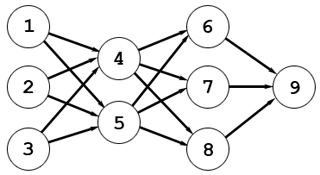시간 제한 메모리 제한 제출 정답 맞은 사람 정답 비율
2 초 256 MB 1 1 1 100.000%

## 문제

An artificial neural network, often called just a neural network, is a mathematical model inspired by biological neural networks. A neural network consists of an interconnected group of artificial neurons, and it processes information using a connectionist approach to computation.

The neural network is called layered if its neurons are organized into groups called layers. There is no connection between two neurons belonging to the same layer.

Let us number the neurons with sequential positive integers from $1$ to $q$, where $q$ is the number of neurons in the network. The connection between neuron $i$ and neuron $j$ can be described with the connection weight $w_{i, j}$.

A neural network can be represented as a directed acyclic graph: neurons can be represented by the vertices, and connections between neurons --- by the edges. The connection weight for each connection can be represented as the weight of the corresponding edge.In the image above there is a neural network consisting of four layers. The first layer contains neurons $1$, $2$ and $3$, the second layer contains neurons $4$ and $5$, the third layer consists of neurons $6$, $7$ and $8$, and the fourth one contains the only neuron $9$.

Each neuron $i$ has its value $v_{i}$, which is calculated by the following formula:

$$v_{i} = \frac{1}{1 + e^{- \sum_{j}^{ } v_{j} \cdot w_{j, i}}}$$

The layered neural network is called binary neural network if it has the following properties:

• For each neuron $i$ of the first layer the value $v_{i}$ is either $0$ or $1$;
• If neuron $a$ belongs to the layer $i$, neuron $b$ belongs to the layer $j$ and $i > j$, then there is no connection from neuron $a$ to neuron $b$. Note that the connection from neuron $b$ to neuron $a$ is still possible;
• The last layer has the only neuron and its value is either $0$ or $1$;
• Each layer contains at least one neuron.

In this problem you are to create a binary neural network that implements the binary function $f(x_{1}$, $x_{2}$, $\ldots$ $x_{n})$ with $n$ arguments.

The first layer of this binary neural network should contain exactly $n$ neurons numbered with sequential positive integers from $1$ to $n$. The value of neuron $i$ will be automatically set to $x_{i}$. All the other neurons should be numbered with sequential positive integers from $n + 1$ to $q$, where $q$ is the number of neurons in the network. All the values $v_{i}$ ($n + 1 \leq i \leq q$) will be calculated by the formula that is given above.

The last layer of this binary neural network should contain the only neuron. The value of this neuron should differ from the value $f(x_{1}$, $x_{2}$, $\ldots$ $x_{n})$ by no more than $10^{-7}$.

The binary network should not contain more than $25$ layers. The number $q$ of neurons should not be greater than $10^{4}$. The total number $e$ of connections should not be greater than $3 \cdot 10^{4}$.

## 입력

The first line of input contains the only integer $n$ ($2 \leq n \leq 10$). The second line of input contains $2^{n}$ characters. Each of these characters is either '0' or '1'. The first character describes the value of $f(0, \ldots, 0, 0)$, the second one describes the value of $f(0, \ldots, 0, 1)$ and so on, the last one describes the value of $f(1, \ldots, 1, 1)$.

## 출력

On the first line output two integers $l$ and $q$ --- the number of layers and the number of neurons in the binary neural network respectively ($2 \leq l \leq 25$, $1 \leq q \leq 10^{4}$).

On the second line output $q$ integers $p_{i}$. Here $p_{i}$ is the number of layer that the neuron $i$ belongs to ($1 \leq p_{i} \leq l$).

On the third line output the only integer $e$ --- the number of connections in the binary neural network ($n \leq e \leq 3 \cdot 10^{4}$).

Each of the following $e$ lines should contain two integers $a_{i}$, $b_{i}$ and one real number $w_{a, b}$ --- description of the connection from $a_{i}$ to $b_{i}$ with the weight $w_{a, b}$ ($|w_{a,b}| \le 1000$).

## 예제 입력 1

3
00010111


## 예제 출력 1

3 7
1 1 1 2 2 2 3
12
1 4 8.906
1 5 0.749
1 6 5.423
2 4 -0.262
2 5 -8.905
2 6 5.582
3 4 -0.663
3 5 1.087
3 6 -12.123
4 7 66.372
5 7 -55.329
6 7 -47.883